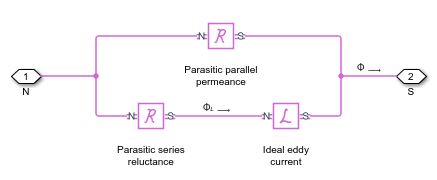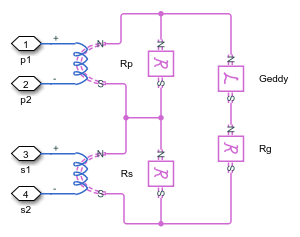# Eddy Current

Eddy current loss representation

•Libraries:
Simscape / Electrical / Passive

## Description

The Eddy Current block models the effect of eddy currents by generating a magnetomotive force (MMF) that opposes changes in the magnetic flux. In addition, the block models parasitic effects using a series reluctance and a parallel leakage permeance, and thermal effects using an optional thermal port, H.

Eddy currents in magnetic core materials are caused by time-varying magnetic fields. These changing field densities induce voltage potentials within the material, causing current to flow in closed loops. Such currents are usually undesired and cause heating of the magnetic material.

Use this block to add eddy current losses in the magnetic domain to a custom transformer or other magnetic component.

The eddy current component is sometimes referred to as magnetic inductance because it is the magnetic-domain analog to an inductor in the electrical domain.

### Equations

This is the equivalent magnetic circuit for the block, including the eddy current path (bottom) and parallel leakage path (top).In the diagram:

• $\Phi$ is the total flux at the terminals. This flux is the summation of the eddy current loop and the parallel leakage path.

• $\Phi$L is the flux through the eddy current loop.

The block calculates the terminal MMF, $\mathcal{F}$, and flux, $\Phi$, as:

`$\begin{array}{c}\mathcal{F}={G}_{\text{eddy}}\frac{d{\Phi }_{L}}{dt}+\mathcal{R}{\Phi }_{L}\\ \Phi =\mathcal{F}\mathcal{P}+{\Phi }_{L}\end{array}$`

where:

• Geddy is the conductance of the eddy current loop.

• $\mathcal{R}$ is the parasitic series reluctance of the eddy current path.

• $\mathcal{P}$ is the parallel permeance.

Because the parasitic series reluctance and parallel permeance are lossless, the total dissipated power over the block is:

`${P}_{diss}={G}_{\text{eddy}}{\left(\frac{d{\Phi }_{L}}{dt}\right)}^{2}$`

### Relating Transformer Electrical and Magnetic Models

In the electrical domain, eddy current losses are modeled using a parallel resistance across the primary winding. This is the equivalent circuit for a nonideal two-winding transformer.In the diagram:

• Lp and Ls are the self-inductances of the primary and secondary windings, respectively.

• Lm is the mutual inductance between the two windings.

• Rm is the mutual resistance between the two windings, caused by the eddy current losses.

This two-winding transformer can similarly be represented in the magnetic domain. This is the equivalent circuit.In the diagram:

• $\mathcal{R}$p and $\mathcal{R}$s are the reluctances associated with the primary and secondary windings, respectively.

• $\mathcal{R}$g is the reluctance associated with the magnetic coupling of the two windings.

• n is the turns ratio between the two windings.

The electrical- and magnetic-domain circuits are equivalent if:

`${\mathcal{R}}_{g}=\frac{{n}^{2}}{{L}_{m}}$`

`${\mathcal{R}}_{p}=\frac{{n}^{2}}{{L}_{p}}$`

`${\mathcal{R}}_{s}=\frac{1}{{L}_{s}}$`

`${G}_{\text{eddy}}=\frac{{n}^{2}}{{R}_{m}}$`

You can use these relationships to calculate the equivalent two-winding transformer properties for one domain from the other.

## Ports

### Conserving

expand all

Magnetic conserving port associated with the north terminal of the block.

Magnetic conserving port associated with the south terminal of the block.

Thermal conserving port associated with the thermal port of the block.

#### Dependencies

To enable this port, select the Show thermal port parameter.

## Parameters

expand all

Conductance, Geddy, of the eddy current loop. This component is the magnetic-domain analog of inductance in the electrical domain.

Parasitic reluctance, $\mathcal{R}$, of the eddy current loop.

Parasitic permeance, $\mathcal{P}$, of the parallel leakage path. To aid simulation convergence in some circuit topologies, set this parameter to a small value.

Whether to enable the thermal port of the block.

Thermal mass of the block. This parameter models the ability of the Eddy Current block to absorb and store thermal energy.

#### Dependencies

To enable this parameter, select the Show thermal port parameter.

 Brown, A. D., J. N. Ross, and K. G. Nichols. "Time-domain simulation of mixed nonlinear magnetic and electronic systems." IEEE Transactions on Magnetics. Vol. 37, Number 1, 2001, pp. 522-532.